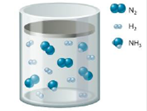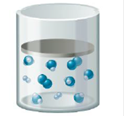Chapter 13, Problem 107CP

Chapter
Section
Textbook Problem

# A mixture of N2, H2, and NH3 is at equilibrium [according to the equation N 2 ( g ) + 3 H 2 ( g ) ⇌ 2 NH 3 ( g ) ] as depicted below:The volume is suddenly decreased (by increasing the external pressure) and a new equilibrium is established as depicted below:a. If the volume of the final equilibrium mixture is 1.00 L, determine the value of the equilibrium constant, K. for the reaction. Assume temperature is constant.b. Determine the volume of the initial equilibrium mixture assuming a final equilibrium volume of 1.00 L and assuming a constant temperature.

(a)

Interpretation Introduction

Interpretation: For the given volume of equilibrium mixture the equilibrium constant and the volume of initial equilibrium mixture is to be calculated.

Concept introduction: The equilibrium constant K describes the ratio of the reactant to the product on the equilibrium conditions in terms of molar concentration.

The equilibrium constant depends upon temperature.

Law of mass action is applicable on the equilibrium reactions.

The Le Chatelier’s principle states that the addition of the reactants shifts the equilibrium to the right while the addition of product shifts the equilibrium to the left at constant temperature.

To determine: The value of equilibrium constant for the given reaction at constant temperature

Explanation

Explanation

Given

The reaction is given as,

N2(g)+3H2(g)2NH3(g)

The volume at equilibrium is 1L .

The given figure clearly indicates that the number of moles Nitrogen, Hydrogen and Ammonia are 4,8 and 2 respectively. After the decrement in volume the new number of moles Nitrogen, Hydrogen and Ammonia are 2,2 and 6 respectively.

The ICE-table for the given equilibrium reaction is stated as,

N2(g)+H2(g)NH3(g)Initialmol482ChangeinmolEquilibriummol226

The above given ICE-table clearly reveals that the change in number of moles of Nitrogen, Hydrogen and Ammonia are 2,6 and 4 respectively.

The value of equilibrium constant is calculated as,

K=[NH3]2[N2][H2]3 (1)

Where,

• K is the equilibrium constant.
• [NH3] is the concentration of NH3 .
• [N2] is the concentration of N2 .
• [H2] is the concentration of H2 .

For the equilibrium moles the concentration is calculated by the formula,

C=nV

Where,

• C is the concentration

(b)

Interpretation Introduction

Interpretation: For the given volume of equilibrium mixture the equilibrium constant and the volume of initial equilibrium mixture is to be calculated.

Concept introduction: The equilibrium constant K describes the ratio of the reactant to the product on the equilibrium conditions in terms of molar concentration.

The equilibrium constant depends upon temperature.

Law of mass action is applicable on the equilibrium reactions.

The Le Chatelier’s principle states that the addition of the reactants shifts the equilibrium to the right while the addition of product shifts the equilibrium to the left at constant temperature.

To determine: The volume of initial equilibrium mixture for the given reaction at constant temperature.

### Still sussing out bartleby?

Check out a sample textbook solution.

See a sample solution

#### The Solution to Your Study Problems

Bartleby provides explanations to thousands of textbook problems written by our experts, many with advanced degrees!

Get Started